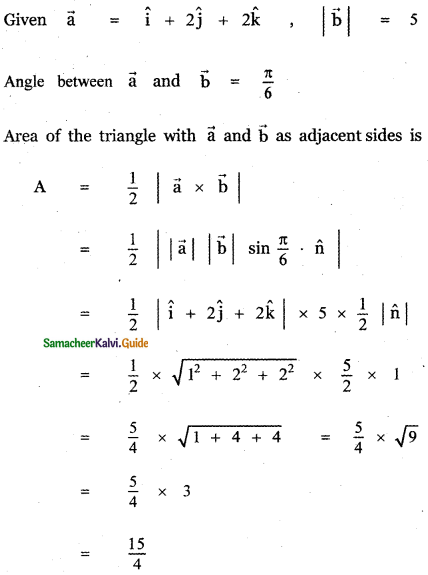Tamilnadu State Board New Syllabus Samacheer Kalvi 11th Maths Guide Pdf Chapter 8 Vector Algebra – I Ex 8.5 Text Book Back Questions and Answers, Notes.

## Tamilnadu Samacheer Kalvi 11th Maths Solutions Chapter 8 Vector Algebra – I Ex 8.5

Choose the correct or the most suitable answer from the given four alternatives:

Question 1.
The value of $$\overrightarrow{\mathbf{A B}}+\overrightarrow{\mathbf{B C}}+\overrightarrow{\mathbf{D A}}+\overrightarrow{\mathbf{C D}}$$ is
(1) $$\overrightarrow{\mathbf{A D}}$$
(2) $$\overrightarrow{\mathbf{C A}}$$
(3) $$\overrightarrow{0}$$
(4) $$-\overrightarrow{\mathbf{A D}}$$
(3) $$\overrightarrow{0}$$

Explaination: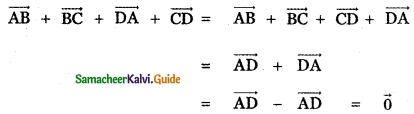Question 2.
If $$\overrightarrow{\mathbf{a}}+2 \overrightarrow{\mathbf{b}}$$ and $$3 \overrightarrow{\mathbf{a}}+\mathbf{m} \overrightarrow{\mathbf{b}}$$ are parallel, then the value of m is
(1) 3
(2) $$\frac{1}{3}$$
(3) 6
(4) $$\frac{1}{6}$$
(3) 6

Explaination: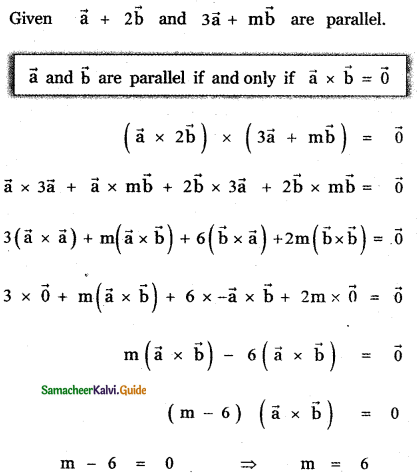Question 3.
The unit vector parallel to the resultant of the vectors î + ĵ – k̂ and î – 2ĵ + k̂ is
(1)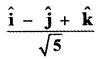(2)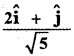(3)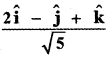(4)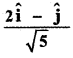(4)Explaination: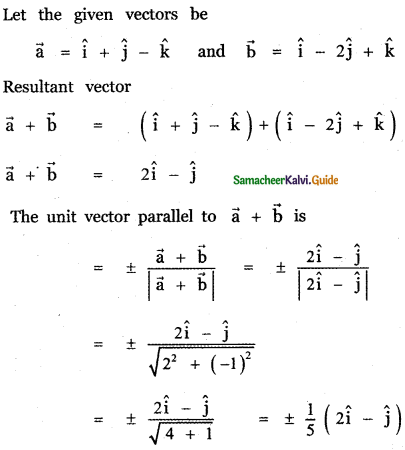Question 4.
A vector $$\overrightarrow{\mathbf{O P}}$$ makes 60° and 45° with the positive direction of the x and y axes respectively. Then the angle between $$\overrightarrow{\mathbf{O P}}$$ and the z – axis is
(1) 45°
(2) 60°
(3) 90°
(4) 30°
(2) 60°

Explaination:
Given the angle made by $$\overrightarrow{\mathbf{O P}}$$ with x – axis and y – axis are 60° and 45° respectively. Let the angle made by $$\overrightarrow{\mathbf{O P}}$$ with the positive direction of z – axis be θ. Then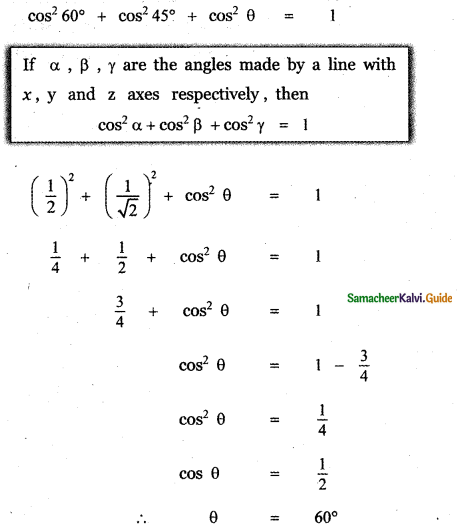Question 5.
If $$\overrightarrow{\mathbf{B A}}$$ = 3î + 2ĵ + k̂ and the position vector of B is î + 3ĵ – k̂ then the position vector A is
(1) 4î + 2ĵ + k̂
(2) 4î + 5ĵ
(3) 4î
(4) – 4î
(2) 4î + 5ĵ

Explaination: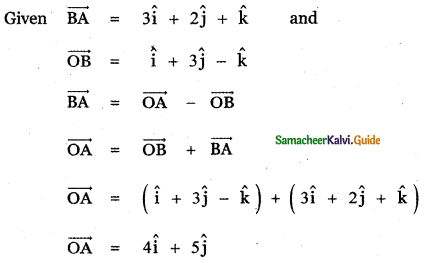Question 6.
A vector makes equal angle with the positive direction of the coordinate axes . Then each angle is equal to
(1) cos-1$$\left(\frac{1}{3}\right)$$
(2) cos-1$$\left(\frac{2}{3}\right)$$
(3) cos-1$$\left(\frac{1}{\sqrt{3}}\right)$$
(4) cos-1$$\left(\frac{2}{\sqrt{3}}\right)$$
(3) cos-1$$\left(\frac{1}{\sqrt{3}}\right)$$

Explaination:
Let the angles made by a vector with the coordinate axes be α, α, α. Then
cos2 α + cos2 α + cos2 α = 1
[If α, β, γ are the angles made by a vector with coordinate axes respectively, then
cos2 α + cos2 β + cos2 γ = 1]
3 cos2 α = 1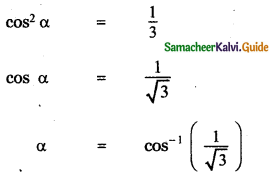Question 7.
The vector $$\overrightarrow{\mathbf{a}}-\overrightarrow{\mathbf{b}}, \overrightarrow{\mathbf{b}}-\overrightarrow{\mathbf{c}}, \overrightarrow{\mathbf{c}}-\overrightarrow{\mathbf{a}}$$ are
(1) parallel to each other
(2) unit vectors
(3) mutually perpendicular vectors
(4) coplanar vectors
(4) coplanar vectors

Explaination: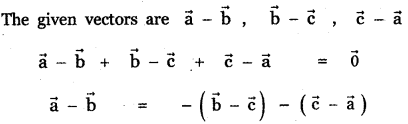[The condition for the three vectors $$\vec{a}$$, $$\vec{b}$$, $$\vec{c}$$ to be coplanar is $$\vec{a}$$ = λ$$\vec{a}$$ + μ$$\vec{b}$$ where λ, μ are scalars. That is one vector is a Linear combination of the other two vectors.]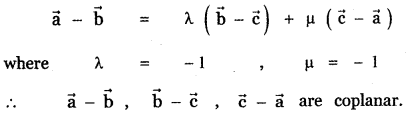Question 8.
If ABCD is a parallelogram, then $$\overrightarrow{\mathbf{A B}}+\overrightarrow{\mathbf{A D}}+\overrightarrow{\mathbf{C B}}+\overrightarrow{\mathbf{C D}}$$ is equal to
(1) 2 $$(\overrightarrow{\mathbf{A B}}+\overrightarrow{\mathbf{A D}})$$
(2) 4 $$\overrightarrow{\mathbf{A C}}$$
(3) 4 $$\overrightarrow{\mathbf{B D}}$$
(4) $$\overrightarrow{0}$$
(4) $$\overrightarrow{0}$$

Explaination: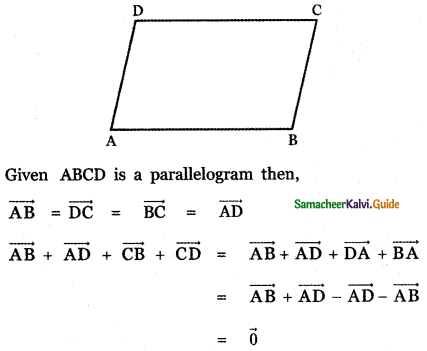Question 9.
One of the diagonals of parallelogram ABCD with $$\vec{a}$$ and $$\vec{b}$$ as adjacent sides is $$\vec{a}$$ + $$\vec{b}$$. The other diagonal BD is
(1) $$\vec{a}$$ – $$\vec{b}$$
(2) $$\vec{b}$$ – $$\vec{a}$$
(3) $$\vec{a}$$ + $$\vec{b}$$
(4) $$\frac{\overrightarrow{\mathbf{a}}+\overrightarrow{\mathbf{b}}}{2}$$
(2) $$\vec{b}$$ – $$\vec{a}$$

Explaination: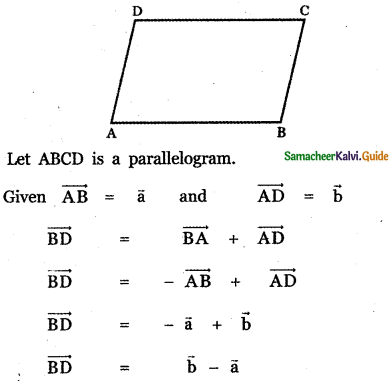Question 10.
If $$\vec{a}$$, $$\vec{b}$$ are the vectors A and B, then which one o the following points whose position vector lies on AB, is
(1) $$\vec{a}$$ + $$\vec{b}$$
(2) $$\frac{2 \vec{a}-\vec{b}}{2}$$
(3) $$\frac{2 \vec{a}+\vec{b}}{3}$$
(4) $$\frac{\vec{a}-\vec{b}}{3}$$
(3) $$\frac{2 \vec{a}+\vec{b}}{3}$$

Explaination: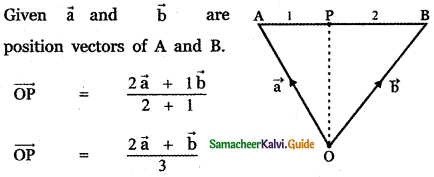Question 11.
If $$\vec{a}$$, $$\vec{b}$$, $$\vec{c}$$ are the position vectors of three collinear points, then which of the following is true?
(1) $$\overrightarrow{\mathbf{a}}=\overrightarrow{\mathbf{b}}+\overrightarrow{\mathbf{c}}$$
(2) $$2 \overrightarrow{\mathbf{a}}=\overrightarrow{\mathbf{b}}+\overrightarrow{\mathbf{c}}$$
(3) $$\overrightarrow{\mathbf{b}}=\overrightarrow{\mathbf{c}}+\overrightarrow{\mathbf{a}}$$
(4) $$4 \overrightarrow{\mathbf{a}}+\overrightarrow{\mathbf{b}}+\overrightarrow{\mathbf{c}}=0$$
(2) $$2 \overrightarrow{\mathbf{a}}=\overrightarrow{\mathbf{b}}+\overrightarrow{\mathbf{c}}$$

Explaination: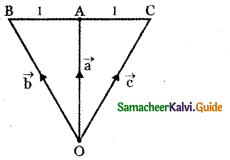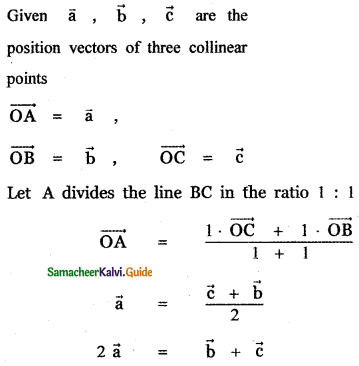Question 12.
If $$\vec{r}$$ = $$\frac{9 \vec{a}+7 \vec{b}}{16}$$, then the point p whose position vector $$\vec{r}$$ divides the line joining the points with position vectors $$\vec{a}$$ and $$\vec{b}$$ in the ratio
(1) 7 : 9 internally
(2) 9 : 7 internally
(3) 9 : 7 externally
(4) 7 : 9 externally
(1) 7 : 9 internally

Explaination: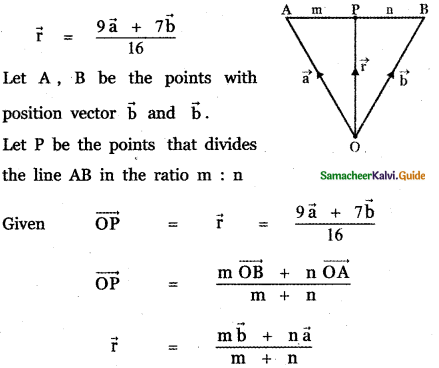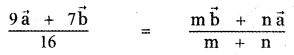Question 13.
If λî + 2λĵ + 2λk̂ is a unit vector, then the value of λ is
(1) $$\frac{1}{3}$$
(2) $$\frac{1}{4}$$
(3) $$\frac{1}{9}$$
(4) $$\frac{1}{2}$$
(1) $$\frac{1}{3}$$

Explaination: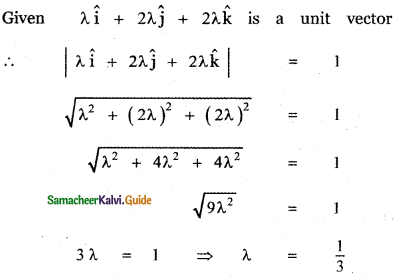Question 14.
Two vertices of a triangle have position vectors 3î + 4ĵ – 4k̂ and 2î + 3ĵ + 4k̂. If the position vector of the centroid is î + 2ĵ + 3k̂, then the position vector of the third vertex is
(1) – 2î – ĵ + 9k̂
(2) – 2î – ĵ – 6k̂
(3) 2î – ĵ + 6k̂
(4) – 2î + ĵ + 6k̂
(1) – 2î – ĵ + 9k̂

Explaination:
Let ABC be a triangle with centroid G. Given that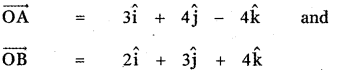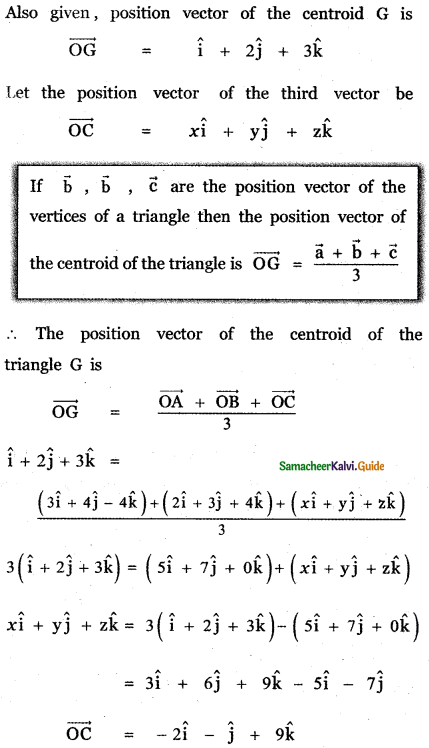Question 15.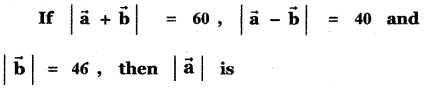(1) 42
(2) 12
(3) 22
(4) 32
(3) 22

Explaination: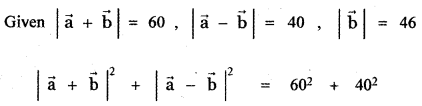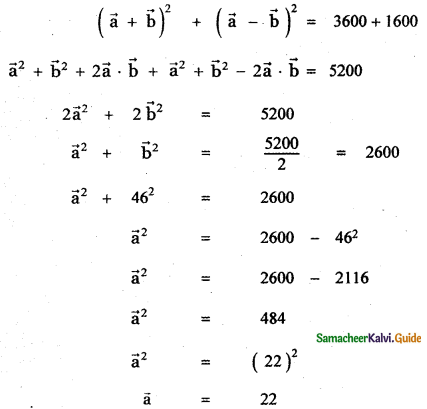Question 16.
If $$\vec{a}$$ and $$\vec{b}$$ having same magnitude and angle between them is 60° and their scalar product $$\frac{1}{2}$$ is then |$$\vec{a}$$| is
(1) 2
(2) 3
(3) 7
(4) 1
(4) 1

Explaination: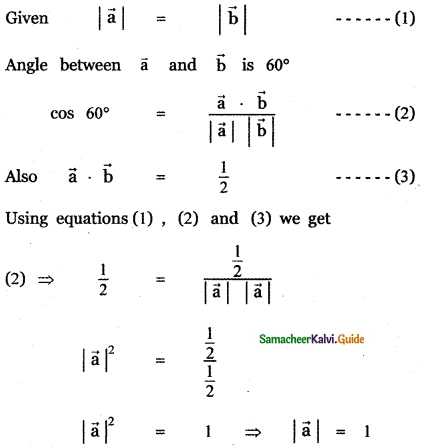Question 17.
The value of θ ∈ (0, $$\frac{\pi}{2}$$) for which the vectors $$\vec{a}$$ = (sin θ) î + (cos θ) ĵ and $$\vec{b}$$ = î – √3ĵ + 2k̂ are perpendicular is equal to
(1) $$\frac{\pi}{3}$$
(2) $$\frac{\pi}{6}$$
(3) $$\frac{\pi}{4}$$
(4) $$\frac{\pi}{2}$$
(1) $$\frac{\pi}{3}$$

Explaination: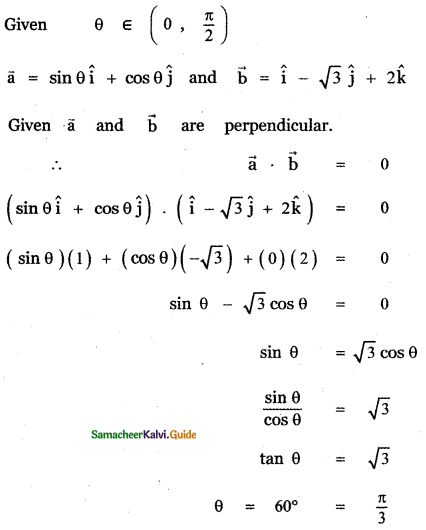Question 18.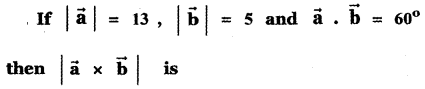(1) 15
(2) 35
(3) 45
(4) 25
(4) 25

Explaination: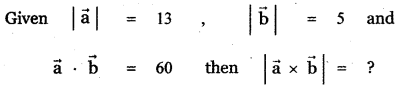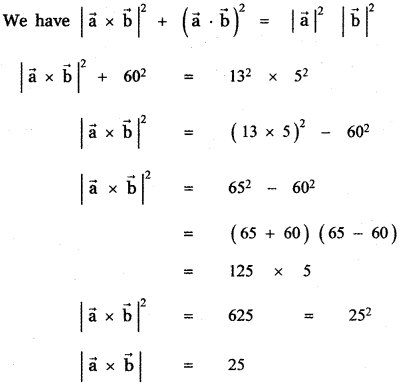Question 19.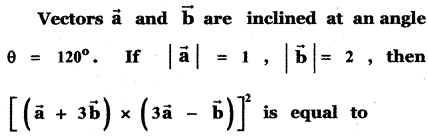(1) 225
(2) 275
(3) 325
(4) 300
(4) 300

Explaination: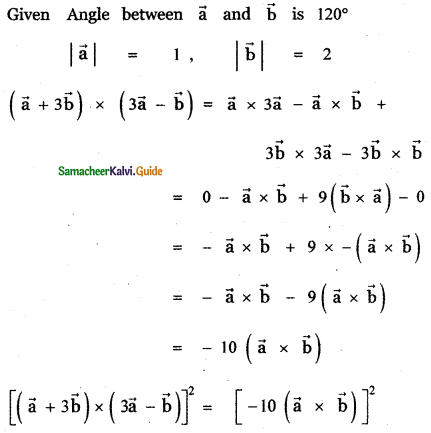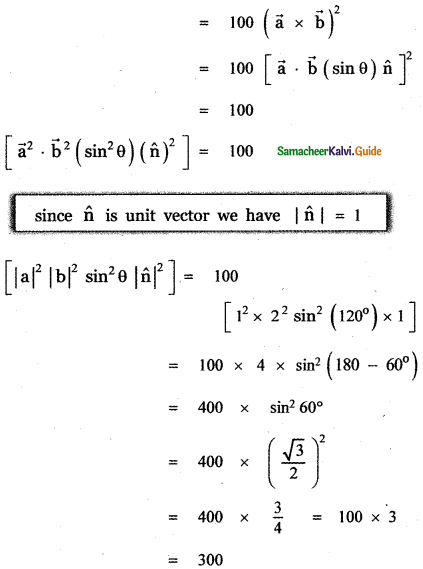Question 20.
If $$\vec{a}$$ and $$\vec{b}$$ are two vectors of magnitude 2 and inclined at an angle 60°, then the angle between $$\vec{a}$$ and $$\vec{a}$$ + $$\vec{b}$$ is
(1) 30°
(2) 60°
(3) 45°
(4) 90°
(1) 30°

Explaination: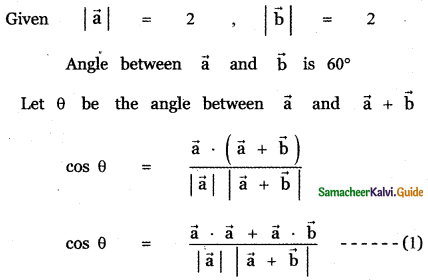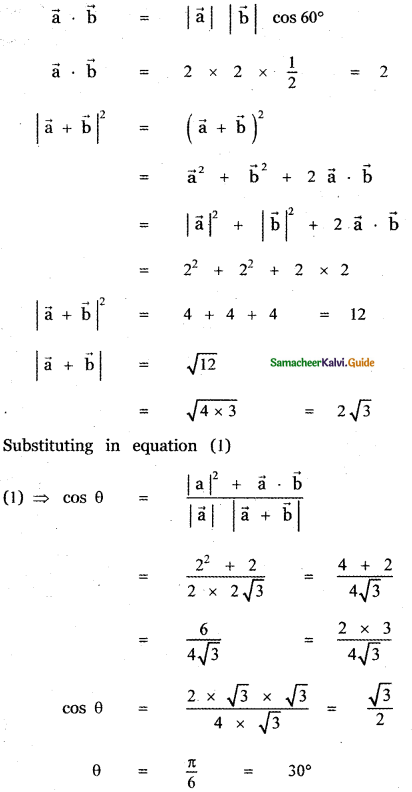Question 21.
If the projection of 5î – ĵ – 3k̂ on the vector î + 3ĵ + λk̂ is same as the projection of î + 3ĵ + λk̂ on 5î – ĵ – 3k̂, then λ is equal to
(1) ± 4
(2) ± 3
(3) ± 5
(4) ± 1
(3) ± 5

Explaination: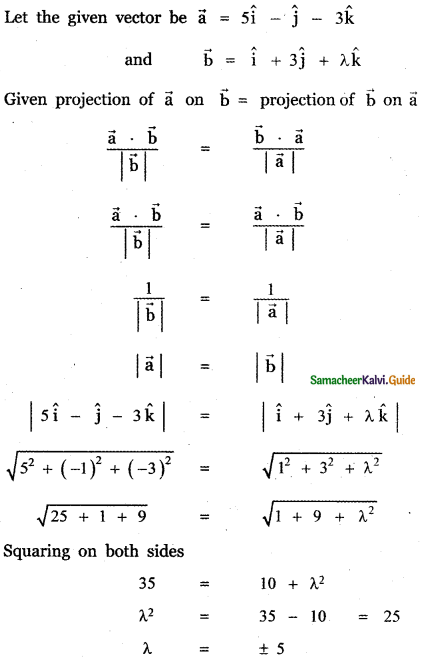Question 22.
If (1, 2, 4) and (2, – 3λ – 3) are the initial and terminal points of the vector î + 5ĵ – 7k̂ then the value of λ is equal to
(1) $$\frac{7}{3}$$
(2) $$-\frac{7}{3}$$
(3) $$-\frac{5}{3}$$
(4) $$\frac{7}{3}$$
(4) $$\frac{7}{3}$$

Explaination: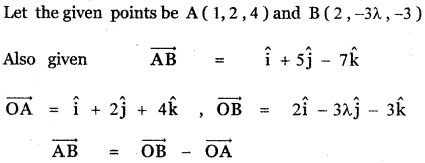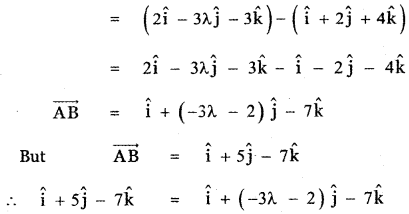Equating the like terms
5 = – 3λ – 2
3λ = – 5 – 2 = – 7
λ = $$-\frac{7}{3}$$Question 23.
If the points whose position vectors 10î + 3ĵ, 12î – 5ĵ and aî + 11ĵ are collinear then a is equal to
(1) 6
(2) 3
(3) 5
(4) 8
(4) 8

Explaination:
The position vectors of the three points are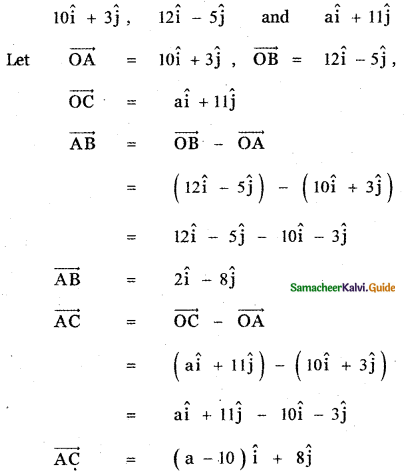The condition for the three points A, B, C are collinear is the area of the triangle formed by these points is zero.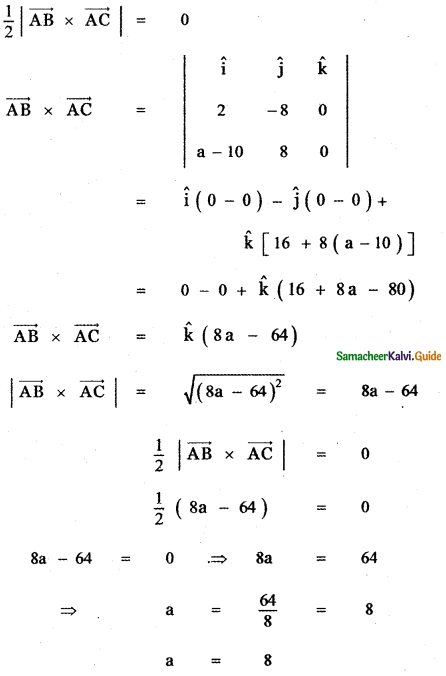Question 24.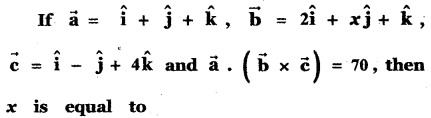(1) 5
(2) 7
(3) 26
(4) 10
(3) 26

Explaination: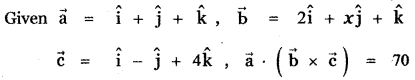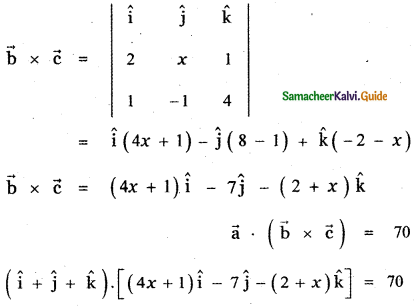(4x + 1) – 7 – (2 + x) = 70
4x + 1 – 7 – 2 – x = 70
3x – 8 = 70
3x = 70 + 8
3x = 78
x = $$\frac{78}{3}$$ = 26Question 25.
If $$\vec{a}$$ = î + 2ĵ + 2k̂, |$$\vec{b}$$| = 5 and the angle between $$\vec{a}$$ and $$\vec{b}$$ is $$\frac{\pi}{6}$$, then the area of the triangle formed by these two vectors as two sides, is
(1) $$\frac{7}{4}$$
(2) $$\frac{15}{4}$$
(3) $$\frac{3}{4}$$
(4) $$\frac{17}{4}$$
(2) $$\frac{15}{4}$$